Author: Oscar Cronquist Article last updated on March 22, 2023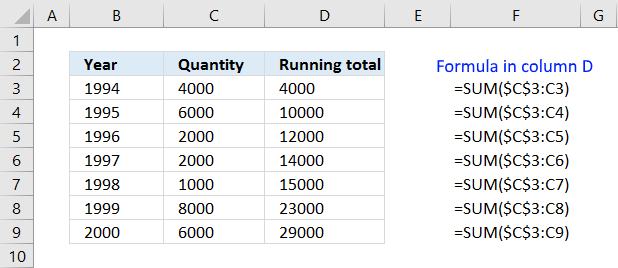This article demonstrates a formula that calculates a running total. A running total is a sum that adds new numbers to the total as you copy the formula to cells below.

The image above shows numbers in column C, the formula in cell D3 adds the number in cell C3 and returns the total which is 4000.

Cell D4 adds the number in cell C3 and cell C4 and returns 10000 which is the result of 4000 + 6000. Cell D5 returns 12000 which is the sum of 4000 + 6000 + 2000 equals 12000.

I also demonstrate running totals formulas that adds values based on a condition, based on month, and when the date changes to a new month.

## 1. Create a formula that returns running totalsThe SUM function in cell D3 uses only a single cell reference and still manages to sum current and previous values in column D. Read on to find out how.

The formula in cell D3:

=SUM(\$C\$3:C3)

### 1.1 Explaining formula

#### Step 1 - Cell reference

\$C\$3:C3

The SUM function has a cell reference that consists of two parts, the first part has a dollar sign before the column character and another one before the row number.

The dollar sign tells you that the cell reference is locked and won't change if you copy the formula. In Excel terminology: an absolute cell reference.

However, the second part of the cell reference has no dollar signs, and that part changes when you copy and paste the formula to other cells. In Excel terminology: a relative cell reference.

What happens when you copy the cell and paste it to cell D4?

=SUM(\$C\$3:C4)

The second part of the cell reference now points to cell C4 and the first part is still pointing to cell C3. The cell reference expands as you copy the formula to cells below.

Recommended articles

How to use absolute and relative references
What is a reference in Excel? Excel has an A1 reference style meaning columns are named letters A to XFD […]

#### Step 2 - Add numbers

The SUM function adds numbers in a cell range or array and returns a total.

SUM(\$C\$3:C4)

becomes

SUM(4000; 6000)

and returns 10000.

## 2. Running totals with a condition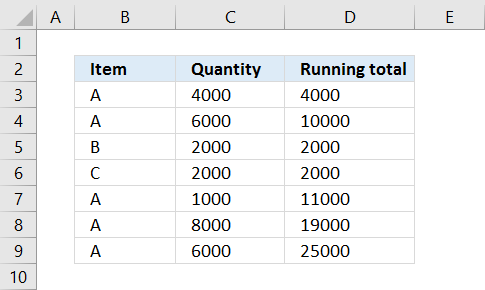The formula above in column D calculates running totals based on a condition. The condition changes depending on the value in column B on the same row as the formula.

Example 1, in cell D6 the formula calculates the sum for Item C. Item C is only in cell B6, the corresponding value in column C is B6. The formula returns 2000 in cell D6.

Example 2, in cell D7 the formula calculates the sum for Item A. Item A is in cell B3, B4 and B7, the corresponding values in column C are C3,C4  and C7.
4000 + 6000 + 1000 = 11000

The formula returns 11000 in cell D7. There are several ways to calculate a running total based on a condition, the easiest and smallest formula is probably the SUMIF function.

Formula in cell D3:

=SUMIF(\$B\$3:B3,B3,\$C\$3:C3)

### 2.1 Explaining formula in cell D9

The arguments in a SUMIF function are: SUMIF(range, criteria, [sum_range])

The range argument grows when the formula is copied to cells below.  \$B\$3:B3 changes to \$B\$3:B4 when the formula is copied to cell D4. This applies to the [sum_range] argument as well.

The expanding cell references make this formula include more and more cells and allowing it to calculate running totals based on a condition.

SUMIF(\$B\$3:B9,B9,\$C\$3:C9)

becomes

SUMIF({"A"; "A"; "B"; "C"; "A"; "A"; "A"},"A", {4000; 6000; 2000; 2000; 1000; 8000; 6000})

and returns 25000. 4000 + 6000 + 1000 + 8000 + 6000 equals 25000.

## 3. Running totals on a monthly basis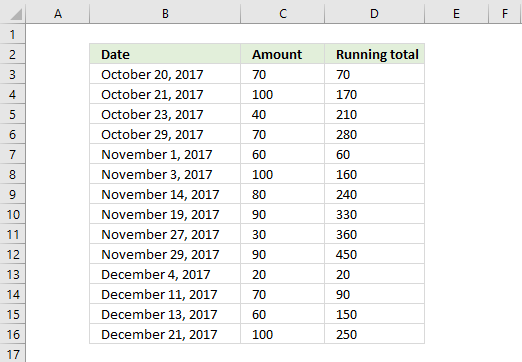This example demonstrates how to create a formula that returns a running total based on the month specified on the same row as the formula.

The dates in column B must be sorted in ascending or descending order for this formula to work appropriately.

Formula in cell D3:

=SUMPRODUCT((TEXT(B3, "YYYY-MM")=TEXT(\$B\$3:B3, "YYYY-MM"))*\$C\$3:C3)

The dates in column B are sorted in ascending order, however, the formula works fine for dates sorted in descending order as well.

The formula in column D adds amounts to a running total using the corresponding date as a condition.

Example, the formula in cell D6 uses this text string "2017-10" in cell B6 as a condition to add all previous amounts above cell D6 that also return text string "2017-10".

In other words, the formula creates a running total for the current month and starts all over when a new month begins.

#### 3.1 Explaining formula in cell D8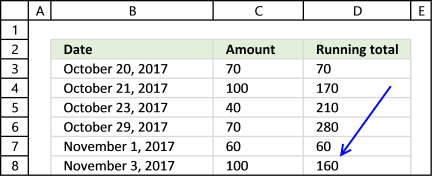Note that I am explaining the formula in cell D8, not cell D3.

#### Step 1 - Convert corresponding date to a text string

TEXT(B8, "YYYY-MM") returns 2017-11

In this case, the TEXT function converts a date to a particular format specified in the second argument. YYYY returns the year and MM returns the month number.

#### Step 2 - Convert corresponding dates to text strings

TEXT(\$B\$3:B8, "YYYY-MM") returns the following array {"2017-10";"2017-10";"2017-10";"2017-10";"2017-11";"2017-11"}

The first argument in the TEXT function is a cell range containing multiple values. The TEXT function returns an array with the exact same number of values.

#### Step 3 - Build a logical expression

TEXT(B8, "YYYY-MM")=TEXT(\$B\$3:B8, "YYYY-MM")

becomes

2017-11={"2017-10";"2017-10";"2017-10";"2017-10";"2017-11";"2017-11"}

and returns {FALSE; FALSE; FALSE; FALSE; TRUE; TRUE}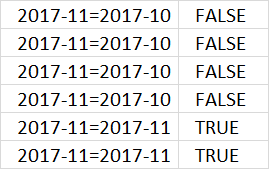#### Step 4 - Multiply with amounts

(TEXT(B8, "YYYY-MM")=TEXT(\$B\$3:B8, "YYYY-MM") )*\$C\$3:C8

becomes

{FALSE; FALSE; FALSE; FALSE; TRUE; TRUE}*{70;170;210;280;60;160;240}

and returns {0; 0; 0; 0; 60; 100}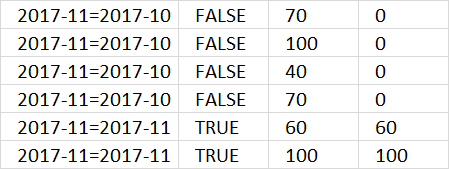#### Step 5 - Sum values

SUMPRODUCT((TEXT(B3, "YYYY-MM")=TEXT(\$B\$3:B3, "YYYY-MM"))*\$C\$3:C3)

becomes

SUMPRODUCT({0; 0; 0; 0; 60; 100})

and returns 160 in cell D8.

Recommended article:

Recommended articles

Running totals based on criteria
Andrew asks: LOVE this example, my issue/need is, I need to add the results. So instead of States and Names, […]

## 4. Calculate a running total when the month changes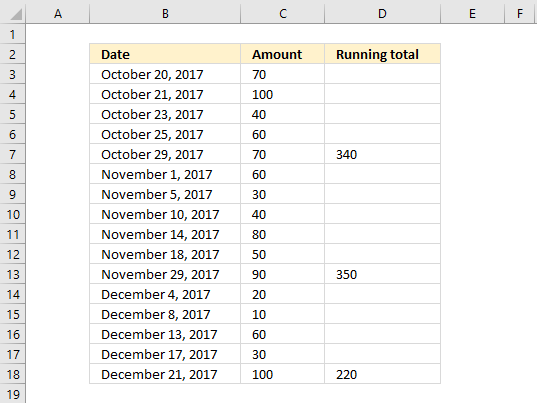Formula in cell D3:

=IF(TEXT(B3, "YYYY-MM")<>TEXT(B4, "YYYY-MM"), SUMPRODUCT(((TEXT(B3, "YYYY-MM"))=(TEXT(\$B\$3:B3, "YYYY-MM")))*\$C\$3:C3), "")

The picture above shows a formula in column D that shows the running total if the next cell in column B contains a new month.

#### Explaining formula in cell D7

Note, I will explain the formula in cell D7. The cell references have changed, the formula now looks like this:

=IF(TEXT(B7, "YYYY-MM")<>TEXT(B8, "YYYY-MM"), SUMPRODUCT(((TEXT(B7, "YYYY-MM"))=(TEXT(\$B\$3:B7, "YYYY-MM")))*\$C\$3:C7), "")

Why will I explain the formula in cell D7? Not much is happening in D3, D4, D5 and D6.

Step 1 - Check if month is not equal to month in cell below

In this example, the TEXT function converts a date to a particular format specified in the second argument. YYYY returns the year and MM returns the month number.

TEXT(B7, "YYYY-MM")<>TEXT(B8, "YYYY-MM")

becomes

"2017-10"<>"2017-11"

and returns TRUE.

Step 2 - Convert corresponding date to a text string

TEXT(B7, "YYYY-MM") returns 2017-10

Step 3 - Convert corresponding dates to text strings

TEXT(\$B\$3:B7, "YYYY-MM") returns the following array {"2017-10";"2017-10";"2017-10";"2017-10";"2017-10"}

The first argument in the TEXT function is a cell range containing multiple values. The TEXT function returns an array with the exact same number of values.

Step 4 - Build a logical expression

TEXT(B7, "YYYY-MM")=TEXT(\$B\$3:B7, "YYYY-MM")

becomes

2017-10={"2017-10";"2017-10";"2017-10";"2017-10";"2017-10"}

and returns {TRUE; TRUE; TRUE; TRUE; TRUE}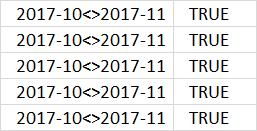Step 5 - Multiply with amounts

(TEXT(B7, "YYYY-MM")=TEXT(\$B\$3:B7, "YYYY-MM") )*\$C\$3:C7

becomes

{TRUE; TRUE; TRUE; TRUE; TRUE}*{70;100;40;60;70}

and returns {70;100;40;60;70}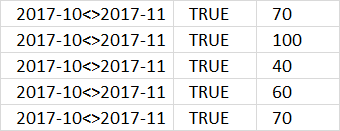Step 6 - Sum values

SUMPRODUCT((TEXT(B7, "YYYY-MM")=TEXT(\$B\$3:B7, "YYYY-MM"))*\$C\$3:C7)

becomes

SUMPRODUCT({70;100;40;60;70})

and returns 340 in cell D8.